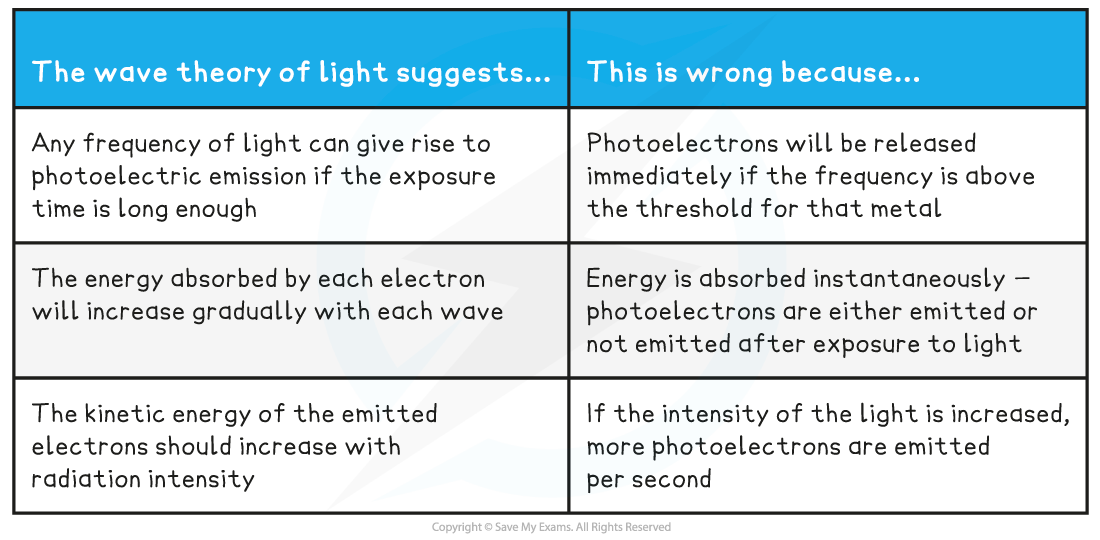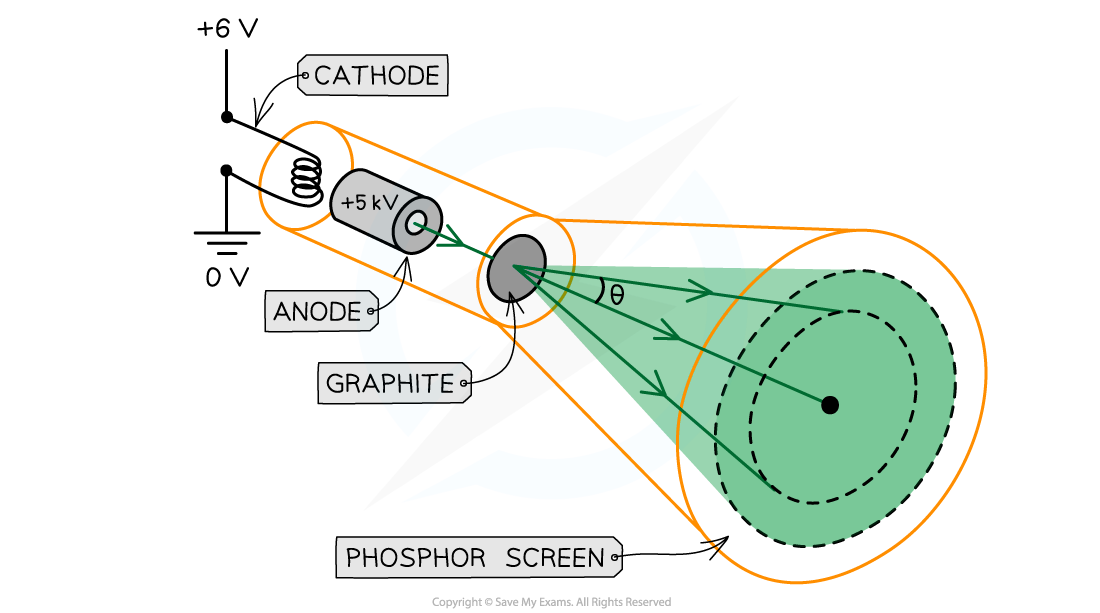# AQA A Level Physics复习笔记2.5.3 Wave-Particle Duality

### Wave-Particle Duality

• Light can behave as a particle (i.e. photons) and a wave
• This phenomenon is called the wave-particle nature of light or wave-particle duality
• Light interacts with matter, such as electrons, as a particle
• The evidence for this is provided by the photoelectric effect

• Light propagates through space as a wave
• The evidence for this comes from the diffraction and interference of light in Young’s Double Slit experiment

#### Light as a Particle

• Einstein proposed that light can be described as a quanta of energy that behave as particles, called photons
• The photon model of light explains that:
• Electromagnetic waves carry energy in discrete packets called photons
• The energy of the photons are quantised according to the equation E = hf
• In the photoelectric effect, each electron can absorb only a single photon - this means only the frequencies of light above the threshold frequency will emit a photoelectron

• The wave theory of light does not support the idea of a threshold frequency
• The wave theory suggests any frequency of light can give rise to photoelectric emission if the exposure time is long enough
• This is because the wave theory suggests the energy absorbed by each electron will increase gradually with each wave
• Furthermore, the kinetic energy of the emitted electrons should increase with radiation intensity
• However, in the photoelectric effect, this is not what is observed

• If the frequency of the incident light is above the threshold and the intensity of the light is increased, more photoelectrons are emitted per second
• Although the wave theory provides good explanations for phenomena such as interference and diffraction, it fails to explain the photoelectric effect

Compare wave theory and particulate nature of light#### Electron Diffraction

• Louis de Broglie discovered that matter, such as electrons, can behave as a wave
• He showed a diffraction pattern is produced when a beam of electrons is directed at a thin graphite film
• Diffraction is a property of waves, and cannot be explained by describing electrons as particlesElectrons accelerated through a high potential difference demonstrate wave-particle duality

• In order to observe the diffraction of electrons, they must be focused through a gap similar to their size, such as an atomic lattice
• Graphite film is ideal for this purpose because of its crystalline structure
• The gaps between neighbouring planes of the atoms in the crystals act as slits, allowing the electron waves to spread out and create a diffraction pattern

• The diffraction pattern is observed on the screen as a series of concentric rings
• This phenomenon is similar to the diffraction pattern produced when light passes through a diffraction grating
• If the electrons acted as particles, a pattern would not be observed, instead, the particles would be distributed uniformly across the screen

• It is observed that a larger accelerating voltage reduces the diameter of a given ring, while a lower accelerating voltage increases the diameter of the rings

#### Investigating Electron Diffraction

• Electron diffraction tubes can be used to investigate the wave properties of electrons
• The electrons are accelerated in an electron gun to a high potential, such as 5000 V, and are then directed through a thin film of graphite
• The electrons diffract from the gaps between carbon atoms and produce a circular pattern on a fluorescent screen made from phosphorExperimental setup to demonstrate electron diffraction

• Increasing the voltage between the anode and the cathode causes the energy, and hence speed, of the electrons to increase
• The kinetic energy of the electrons is proportional to the voltage across the anode-cathode:

Ek = ½ mv2 = eV